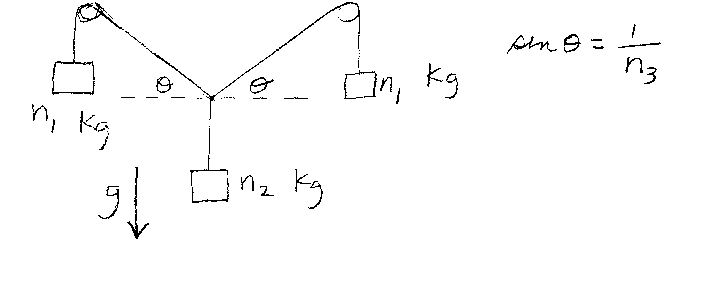Problem A2: In the figure above, the three weights are hanging in static equilibrium. The two weights left and right of center have a mass of n1 Kg. The weight in the center has a mass of n2 Kg. Treat the strings as massless and the pulleys as frictionless. If the sin of the angle &theta in the figure is sin &theta = 1/n3 , what is n3?n1 = n2 = Input n3:

If you are currently in my class, you can record your grade by entering your name and student ID number (without the leading zeros) below and clicking on "record grade".
 First Name = Last Name = ID = Problem: